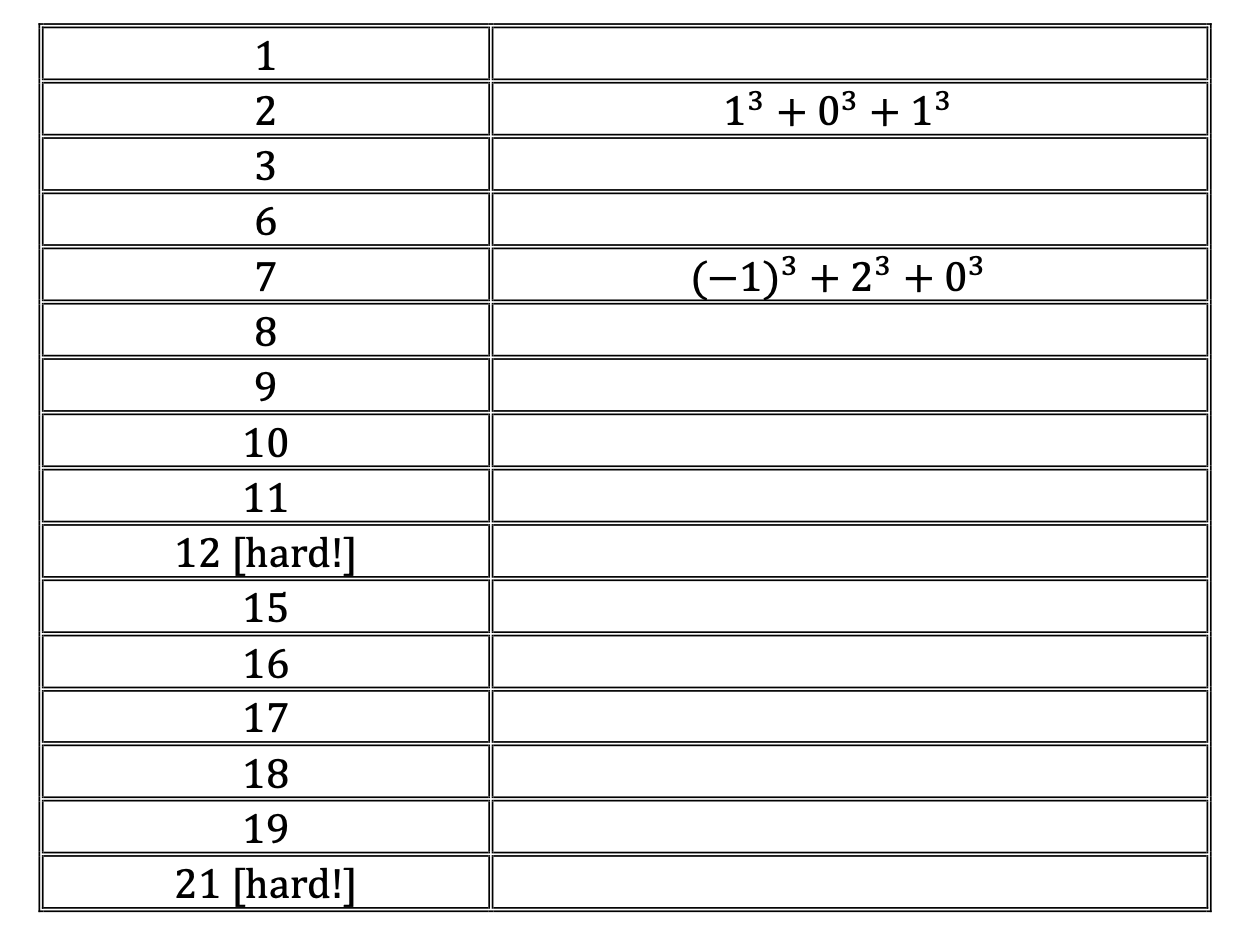# Week 6: Sum of 3 Cubes

Week 6: Sum of 3 Cubes

(a) How many of the following numbers in the table can you form as the sum of 3 cubes of integers?  (You can use negative integers if you wish).(b)  Research what the solution is for 30 and 33!

(c)  Can you find a pattern that describes the numbers that are missing from this list?

Teacher notes:

Level of difficulty: Accessible for all

Syllabus knowledge required: None

This would be a good starter which could either be done when studying proof in Analysis.